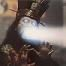# Gaming Quiz / Hearthstone: Best and Worst Stats Minions (Karazhan)

Random Gaming Quiz

## Can you name the Hearthstone minions with the best and worst stats per mana? See note*

#### bysmuglapse  Plays Quiz not verified by Sporcle

Score
0/55
Timer
17:00
Best Values (Stats/Mana > 3)
6/1 = 6
5/1 = 5
5/1 = 5
9/2 = 4.5
4/1 = 4
4/1 = 4
4/1 = 4
4/1 = 4
8/2 = 4
4/1 = 4
4/1 = 4
4/1 = 4
4/1 = 4
4/1 = 4
4/1 = 4
4/1 = 4
11/3 = 3.67
7/2 = 3.5
14/4 = 3.5
14/4 = 3.5
7/2 = 3.5
7/2 = 3.5
7/2 = 3.5
10/3 = 3.33
10/3 = 3.33
16/5 = 3.2
Worst Values (Stats/Mana ≤ 1)
2/4 = 0.5
2/4 = 0.5
2/4 = 0.5
16/25 = 0.64
2/3 = 0.67
5/6 = 0.83
6/7 = 0.86
8/9 = 0.89
8/8 = 1
3/3 = 1
6/6 = 1
3/3 = 1
1/1 = 1
2/2 = 1
2/2 = 1
3/3 = 1
4/4 = 1
2/2 = 1
3/3 = 1
2/2 = 1
4/4 = 1
2/2 = 1
3/3 = 1
6/6 = 1
2/2 = 1
2/2 = 1
2/2 = 1
5/5 = 1
4/4 = 1

## From the Vault See Another

You've got to commend the writers for making a bunch of 30-somethings celebrating the end of high school look convincing.

### Extras

Created Feb 10, 2016